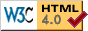# Line Height Inheritance Test and Line Box Test

This is a variant of a test by Ian Hickson.

Paragraphs one and four should show the same behavior. Paragraphs two and three should show the same behavior.

To go beyond an inheritance test, that behavior should be the following. In tests one and four, the large text should cause additional spacing above the line in which it is contained, but not below. The distance from the small text in the line containing the big text to the small text in the line below should be the same as any of the other such distances (except the one above the big text. In paragraphs two and three, the big text should add extra space to both top and bottom.

Calculated line height here should be 13 pixels. Calculated line height here should be 13 pixels. Calculated line height here should be 13 pixels. Calculated line height here should be 13 pixels. Calculated line height here should be 13 pixels. Calculated line height here should be 13 pixels. Calculated line height here should be 13 pixels. Calculated line height here should be 13 pixels. Calculated line height here should be 13 pixels. (big text) Calculated line height here should be 13 pixels. Calculated line height here should be 13 pixels. Calculated line height here should be 13 pixels. Calculated line height here should be 13 pixels. Calculated line height here should be 13 pixels. Calculated line height here should be 13 pixels. Calculated line height here should be 13 pixels. Calculated line height here should be 13 pixels.

Calculated line height here should be 13 pixels. Calculated line height here should be 13 pixels. Calculated line height here should be 13 pixels. Calculated line height here should be 13 pixels. Calculated line height here should be 13 pixels. Calculated line height here should be 13 pixels. Calculated line height here should be 13 pixels. Calculated line height here should be 13 pixels. Calculated line height here should be 13 pixels. (big text) Calculated line height here should be 13 pixels. Calculated line height here should be 13 pixels. Calculated line height here should be 13 pixels. Calculated line height here should be 13 pixels. Calculated line height here should be 13 pixels. Calculated line height here should be 13 pixels. Calculated line height here should be 13 pixels. Calculated line height here should be 13 pixels.

Calculated line height here should be 13 pixels. Calculated line height here should be 13 pixels. Calculated line height here should be 13 pixels. Calculated line height here should be 13 pixels. Calculated line height here should be 13 pixels. Calculated line height here should be 13 pixels. Calculated line height here should be 13 pixels. Calculated line height here should be 13 pixels. Calculated line height here should be 13 pixels. (big text) Calculated line height here should be 13 pixels. Calculated line height here should be 13 pixels. Calculated line height here should be 13 pixels. Calculated line height here should be 13 pixels. Calculated line height here should be 13 pixels. Calculated line height here should be 13 pixels. Calculated line height here should be 13 pixels. Calculated line height here should be 13 pixels.

Calculated line height here should be 13 pixels. Calculated line height here should be 13 pixels. Calculated line height here should be 13 pixels. Calculated line height here should be 13 pixels. Calculated line height here should be 13 pixels. Calculated line height here should be 13 pixels. Calculated line height here should be 13 pixels. Calculated line height here should be 13 pixels. Calculated line height here should be 13 pixels. (big text) Calculated line height here should be 13 pixels. Calculated line height here should be 13 pixels. Calculated line height here should be 13 pixels. Calculated line height here should be 13 pixels. Calculated line height here should be 13 pixels. Calculated line height here should be 13 pixels. Calculated line height here should be 13 pixels. Calculated line height here should be 13 pixels.(Back to CSS Testing Information, David Baron)